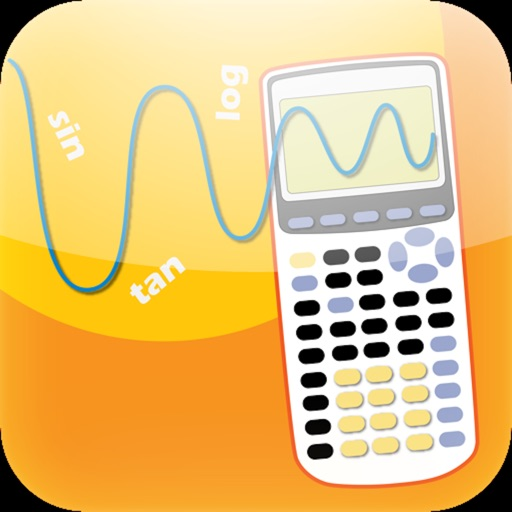## It is the scientific graphing calculator which can plot 2D and 3D graph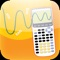# Graphing Calculator 3D

by Kejian Jin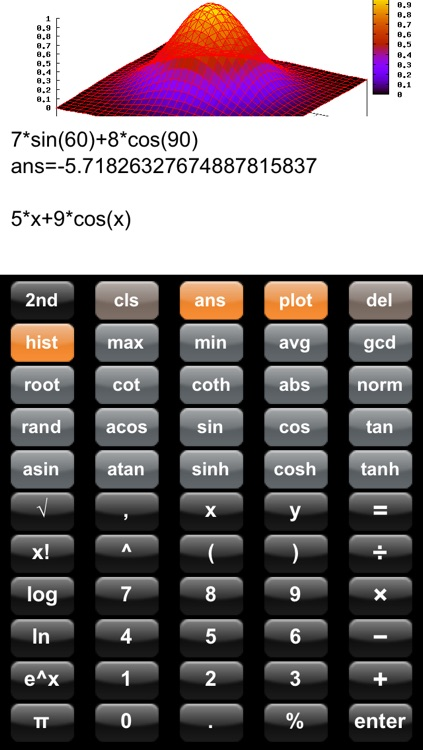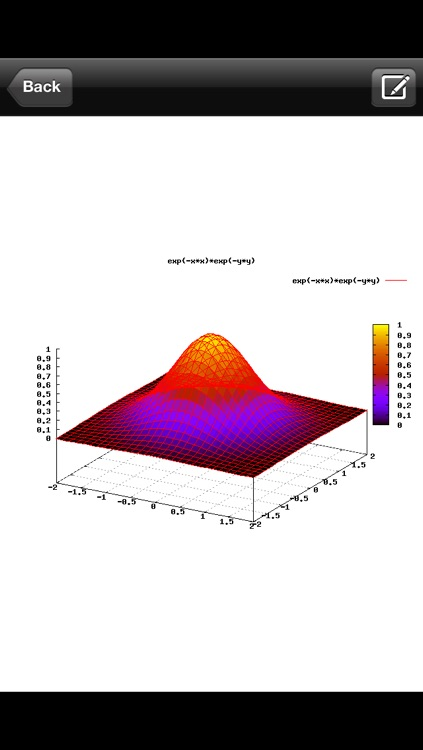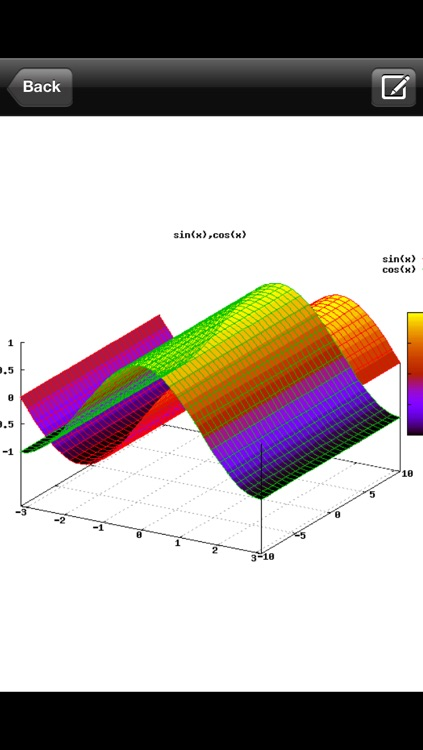It is the scientific graphing calculator which can plot 2D and 3D graph. It is similar to Texas Instruments TI-83, TI-84. It has more features such as plotting 3D graph for mathematics functions.### App Details

Version
2.8
Rating
(581)
Size
3Mb
Genre
Education Utilities
Last updated
November 21, 2015
Release date
September 26, 2009

### App Screenshots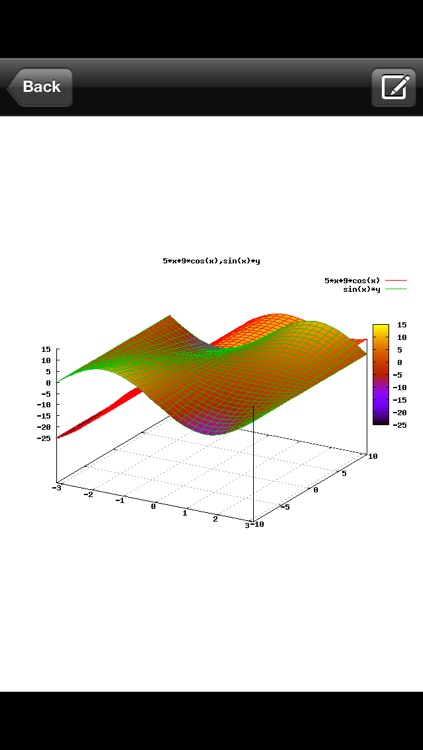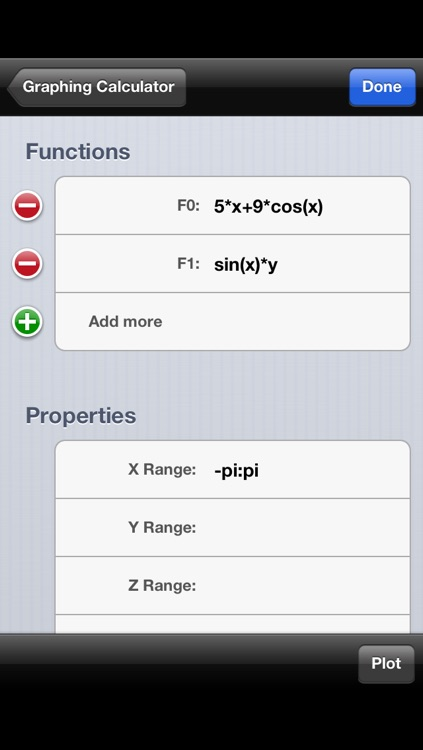### App Store Description

It is the scientific graphing calculator which can plot 2D and 3D graph. It is similar to Texas Instruments TI-83, TI-84. It has more features such as plotting 3D graph for mathematics functions.

News: This app supports iPad natively with full width and height.

Features:
- plot 2D and 3D graph
- plot multiple functions on one graph
- any plot function will be saved and can be edited and plotted later.
- send email with the graph after you done.
- easily zoom in and out on the graph
- can operate on very large number mathematics.
- convert number to any base
- compute permutation, combination - compute greatest common denominator
- compute least common multiple of a set of rational numbers
- compute factorial and fibonacci number.
- provide many trigonometric functions
- calculate the norm of value
- compute matrix determinant, inverse matrix.
- stream line interface so you can easily change the input with finger.
- copy/paste your result to any other application
- provide many other scientific functions
- build-in Help manual for user.

After you use this graphing calculator, you will never want to use any other graphing calculator. It is very simple and easy to use.

Functions in scientific graphing calculator:

= it can plot f(x), f(x,y)

= The following math functions you can use to plot 2D and 3D Graph:
- abs, norm, rand, acos,
- sin, cos, tan, asin, atan,
- sinh, cosh, tanh, log, ln, exp, sqrt,
- any linear, quadratic or x to n, exponential functions.

= The following math functions you can use for calculation:
- max, min, avg, gcd, root, cot, coth
- asinh, acosh, atanh, base, perm, comb,
- ceil, floor, round, lcm, fib

= The following function use to compute matrix
- determinant: det(x)
- inverse matrix: inverse(x)

To start calculate, simply enter:

7+30*6

To Plot a linear function, simply enter:

13*x+6

Click "Plot" button. You do not need enter "y=" in the front.
It is wrong if you enter: y=13*x+6 because it assumed it is a function of x or y or both. It is also wrong if you enter 13(x)+6. For example, if you want to plot f(x,y)=3*x+4*y, you only need input the second part. Note that you need math operator for any math formula.

To access "Help" document. Click button "Hist" and click "Help.

It is highly recommended that you read the "Quick Start Guide" in build-in Help document before use this application.

Disclaimer:
AppAdvice does not own this application and only provides images and links contained in the iTunes Search API, to help our users find the best apps to download. If you are the developer of this app and would like your information removed, please send a request to [email protected] and your information will be removed.